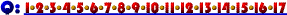12. Suppose a manufacturing firm learns that its annual property tax payments will decrease. We would depict this by:

1. shifting the average fixed cost, the marginal cost, and the average total cost curves up.
2. shifting the average total, the average fixed, and the average variable cost curves down.
3. shifting the marginal cost, the average variable cost, and the average fixed cost curves down.
4. shifting the marginal cost, the average total cost, and the average fixed cost curves down.
5. shifting the average total, and the average fixed cost curves downward.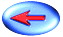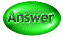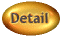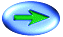Click Here if you don't see topics listed to the left and below.